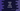# 6 ways to add items to a list in Kotlin## How to add items to a list in Kotlin:

This post will show you how to add items to a list in Kotlin. We can add single or multiple items to a Kotlin list easily. We need one Mutable list for that. The examples we are showing in this post can also be used with custom object lists.

### Adding a single element using add():

add is used to add one single element to a list. This method is defined as below:

``fun add(element: E): Boolean``

It adds the element to the list and returns one boolean value defining the addition is a success or not.

Also, another add method is available to insert an element at a specific index:

``fun add(index: Int, element: E): Unit``

It takes the index as the first parameter.

Let’s take a look at the example below:

``````fun main() {
val givenList = mutableListOf("one", "two", "three")

println(givenList)

println(givenList)
}``````

Here, I am adding only one item to the mutable list each time. First time, we are not passing the index and in the second time, we are passing the index as 0. It prints the below output:

``````[one, two, three, four]
[zero, one, two, three, four]``````

The first add inserts the item at the end and the second add inserts the item in the beginning.

addAll works similar to add. We can use this method to add more than one values. Following are the definitions of this method:

``````public fun addAll(index: Int, elements: Collection<E>): Boolean

override fun addAll(elements: Collection<E>): Boolean``````

As you can see here, we can provide an index and a collection to insert it from a specific index or we can directly insert the items at the end.

``````fun main() {
val givenList = mutableListOf("one", "two", "three")
givenList.addAll(0,arrayListOf("-3", "-2", "-1", "0"))

println(givenList)
}``````

This example, adds the arraylist at index 0. It modifies the mutable list givenList.

It will print the below output:

``[-3, -2, -1, 0, one, two, three]``

### Adding items using plus() and plusElement():

Using plus and plusElement, we can add single or multiple items to a given list. But, it doesn’t change the original list. It creates a new list and returns that list.

``````fun main() {
val givenList = mutableListOf("one", "two", "three")
val newList = givenList.plus("four").plus("five").plus(arrayOf("six", "seven", "eight"))

println(newList)

val anotherList = givenList.plusElement("nine")
println(anotherList)
}``````

It is not changing the original list givenList. So, we are defining new variables to hold the result.

It prints the below output:

``````[one, two, three, four, five, six, seven, eight]
[one, two, three, nine]``````

### Using plusAssign():

plusAssign modifies the mutable list. This method is similar to plus, but it doesn’t return a new list. It directly changes the original list.

For example:

``````fun main() {
val givenList = mutableListOf("one", "two", "three")
givenList.plusAssign("four")
givenList.plusAssign("five")
givenList.plusAssign(arrayOf("six", "seven", "eight"))

println(givenList)
}``````

It prints :

``[one, two, three, four, five, six, seven, eight]``

### Using + operator:

+ works similar to plus. It creates a new list without modifying the original list. For example:

``````fun main() {
val givenList = mutableListOf("one", "two", "three")
val newList = givenList + "four" + "five" + "six"

println(newList)
}``````

It gives the below output:

``[one, two, three, four, five, six]``